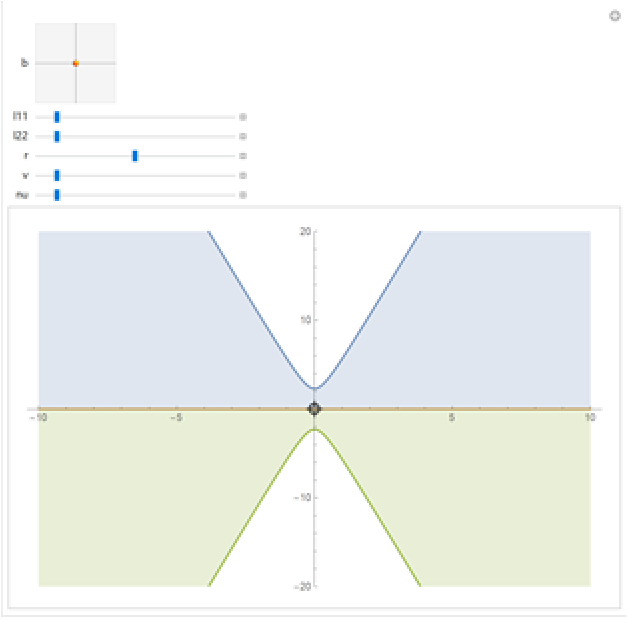#Function Repository Resource:

# BayesianLinearRegression

Perform Bayesian linear regression with conjugate priors

Contributed by: Sjoerd Smit
 ResourceFunction["BayesianLinearRegression"][{{var11,var12,…,y1},{var21,var22,…,y2},…},{f1,f2,…},{var1,var2,…}] performs a Bayesian linear model fit on the data using basis functions fi with independent variables vari. ResourceFunction["BayesianLinearRegression"][{{var11,var12,…}→y1,{var21,var22,…}→y2,…},…] generates the same result. ResourceFunction["BayesianLinearRegression"][{{var11,var12,…},{var21,var22,…},…}→{y1,y2,…},…] generates the same result.

## Details and Options

The yi in the input data can be either scalar or vector observations.
The number of vari have to match the dimensions of the inputs xi.
ResourceFunction["BayesianLinearRegression"] inherits the IncludeConstantBasis option from DesignMatrix. The default option value is "IncludeConstantBasis"True.
By default, a weakly informative prior is assumed that is still normalizable so that the log-evidence is well-defined. Different priors can be specified with the "PriorParameters" option in the same format as the output of ResourceFunction["BayesianLinearRegression"] (see options section below).
The output of ResourceFunction["BayesianLinearRegression"] is an association containing the following items:
 “PriorParameters” Values of the hyperparameters corresponding to the prior distribution of the fit coefficients. “PosteriorParameters” Values of the hyperparameters corresponding to the posterior distribution of the fit coefficients. “LogEvidence” The natural log of the marginal likelihood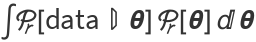, which is the Bayesian goodness-of-fit measure of the model. “Prior” Prior distributions. “Posterior” Posterior distributions. “Basis” The basis functions supplied by the user. “IndependentVariables” The independent variables supplied by the user. “Functions” Functions that yield distributions when applied arbitrary hyperparameters. Only included when the "IncludeFunctions"→ True option is set.
The keys "Prior", "Posterior" and "Functions" contain the following items:
 “PredictiveDistribution” Predictive distribution of the data. “UnderlyingValueDistribution” The distribution of of the fit line of the data. “RegressionCoefficientDistribution” Marginal distribution of the fit coefficients. “ErrorDistribution” Marginal distribution of the variance (for 1D regression) or covariance matrix.
The implementation of the formulas is based on the Wikipedia article on multivariate Bayesian linear regression (see link below). Univariate regression (i.e., when the yi are scalars or 1D vectors) is treated as a special case of multivariate regression using the lower-dimensional equivalents of the multivariate and matrix distributions.

## Examples

### Basic Examples

Generate a Bayesian linear regression on random data:

 In:=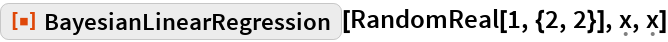Out=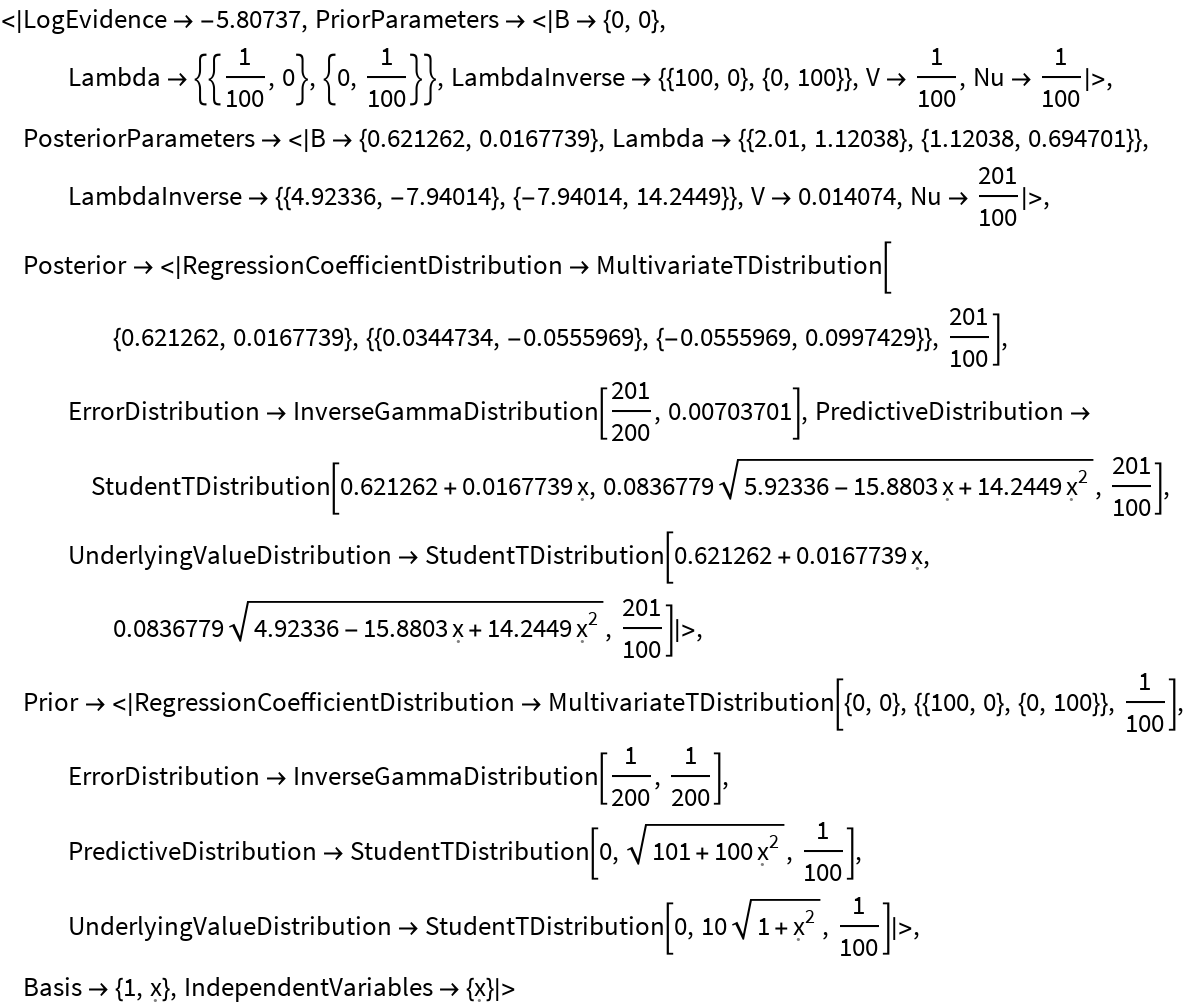Generate test data:

 In:=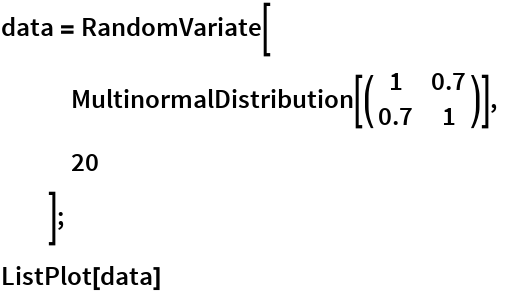Out=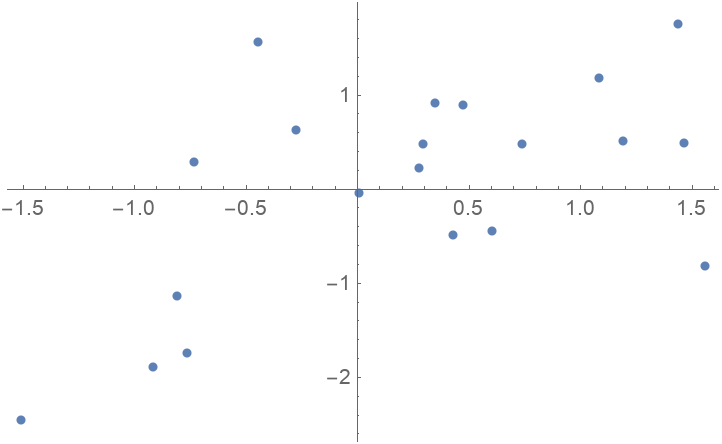Fit the data with a 1st order polynomial:

 In:=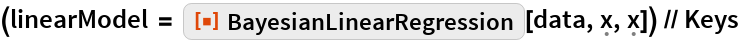Out=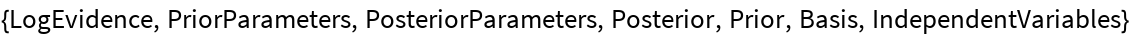Show the predictive distribution of the model and the distribution of the fit line (dashed):

 In:=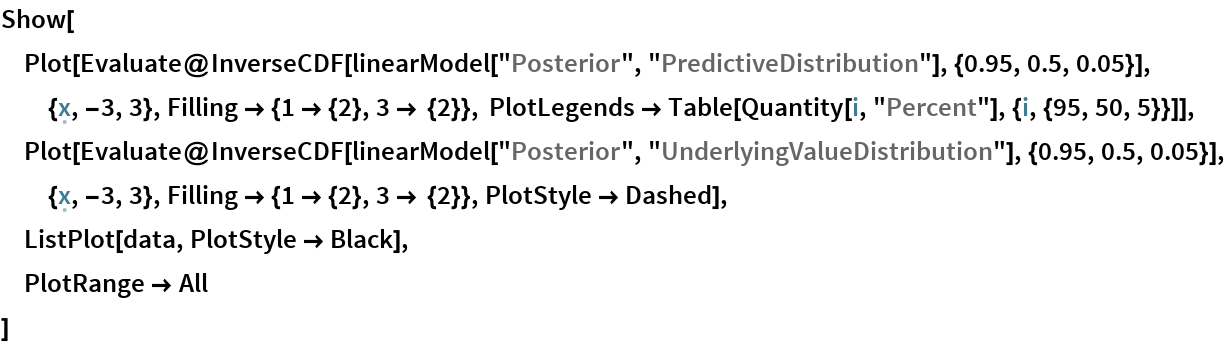Out=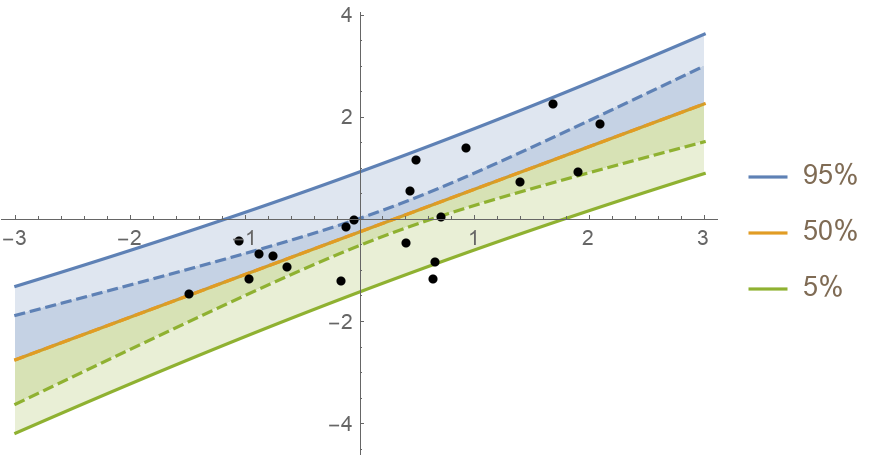Plot the joint distribution of the coefficients a and b in the regression equation y==ax+b:

 In:=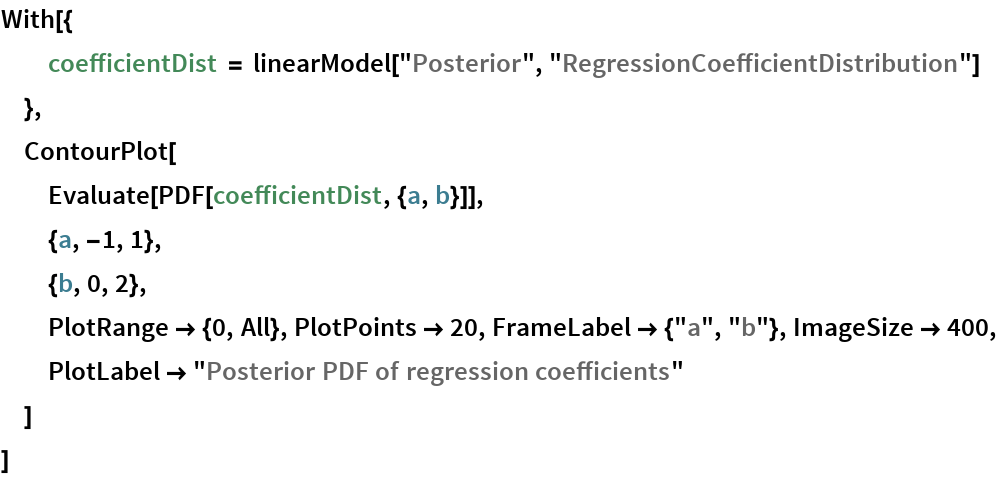Out=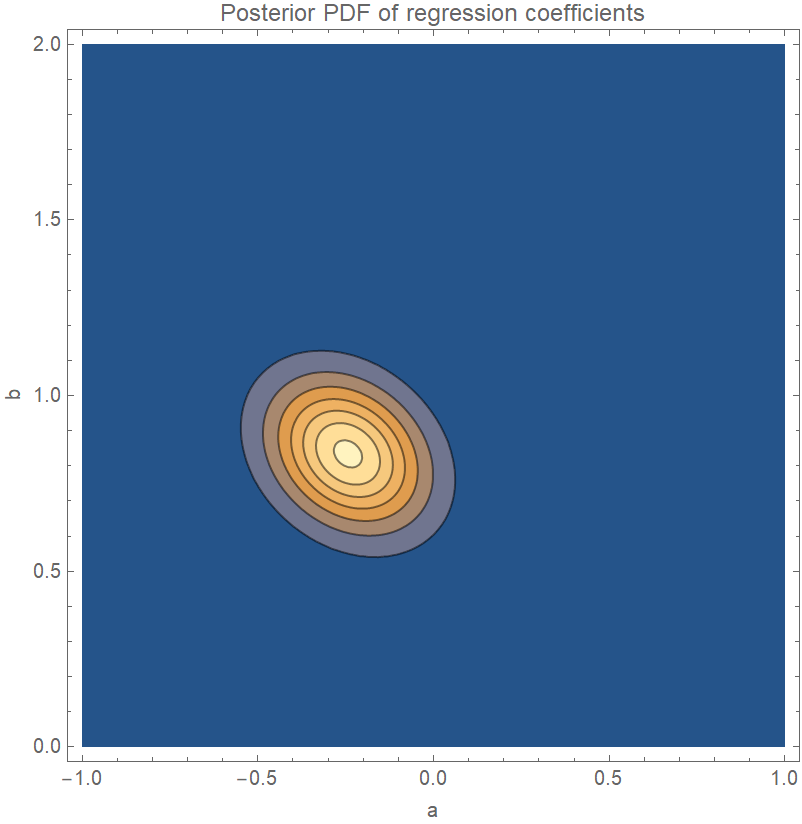Plot the pdf of the posterior variance of the residuals:

 In:=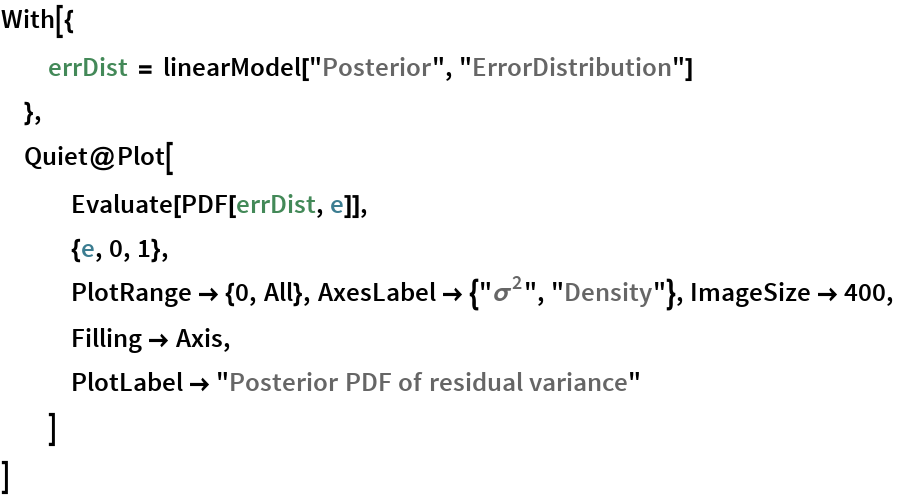Out=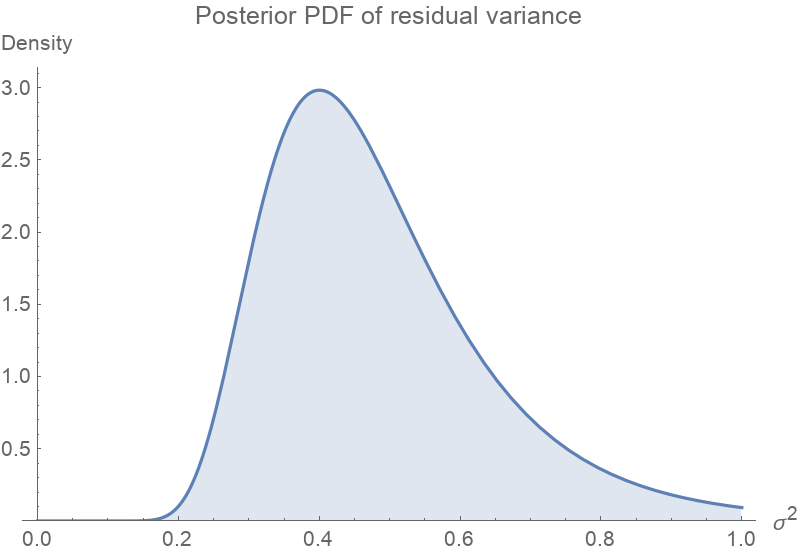Fit the data with a polynomial of arbitrary degree and compare the prediction bands and log-evidence of fits up to degree 4:

 In:=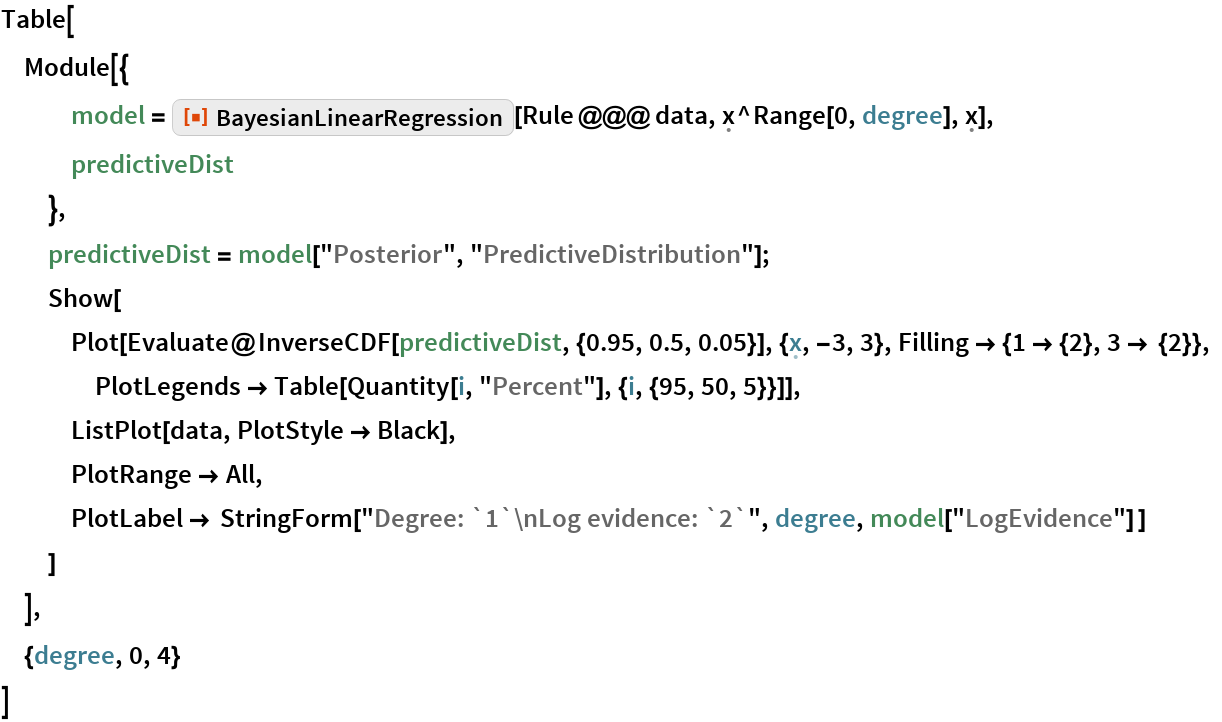Out=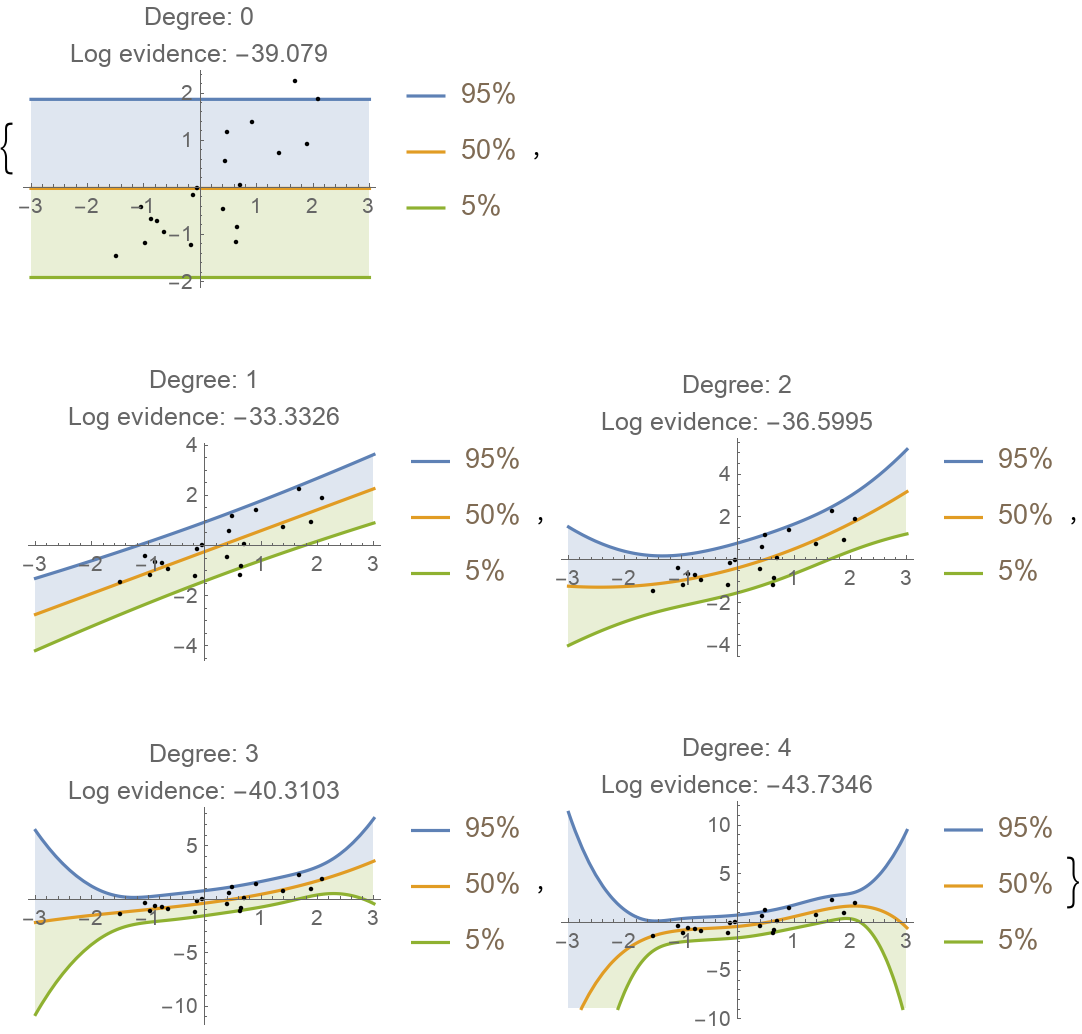### Scope

BayesianLinearRegression can do multivariate regression. For example, generate some 3D data:

 In:=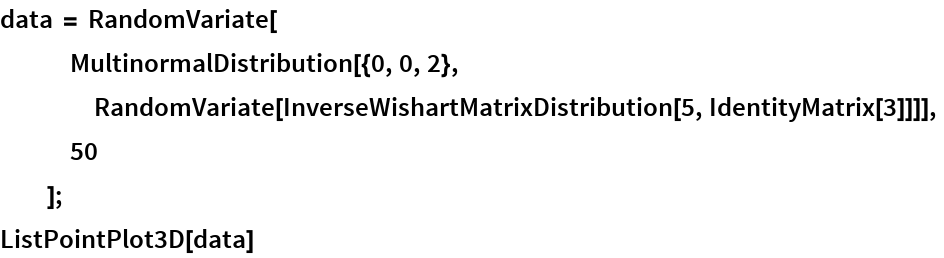Out=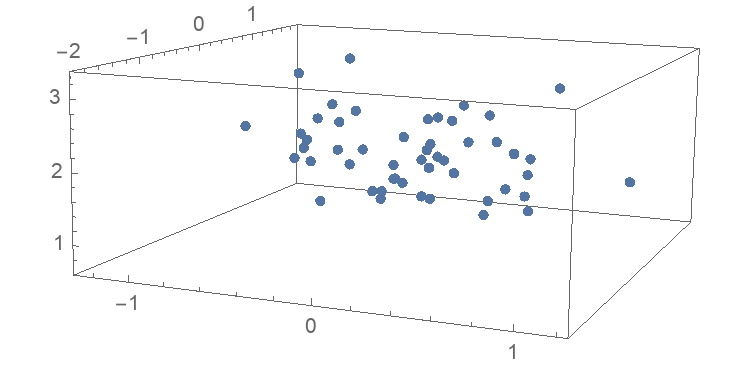Do a regression where the x coordinate is treated as the independent variable and y and z are both dependent on x:

 In:=Out=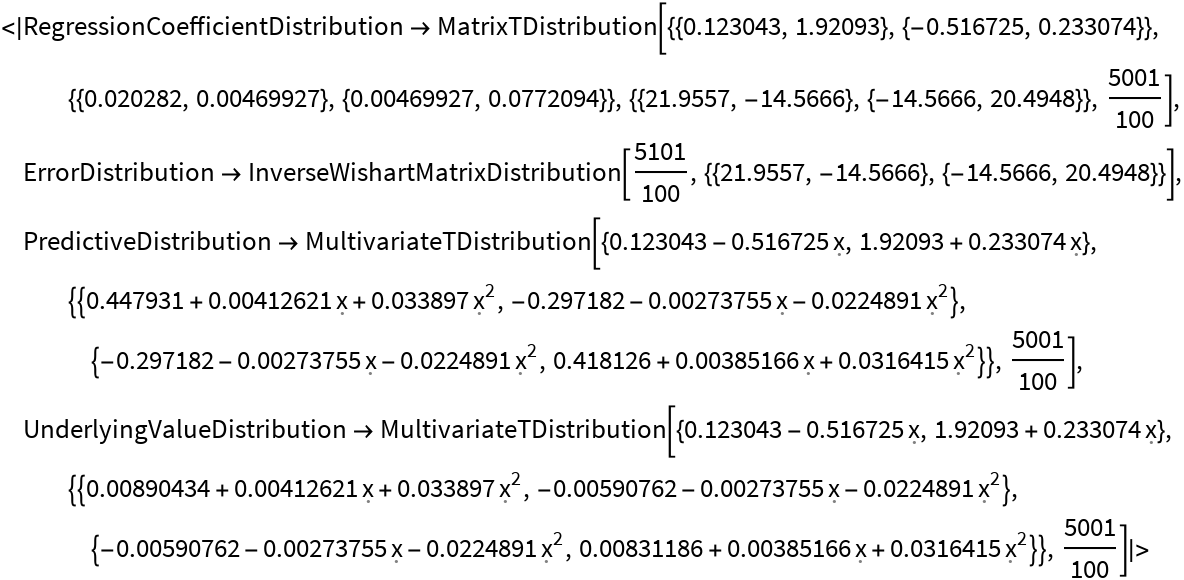The predictive joint distribution for y and z is a MultivariateTDistribution:

 In:=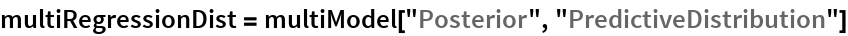Out=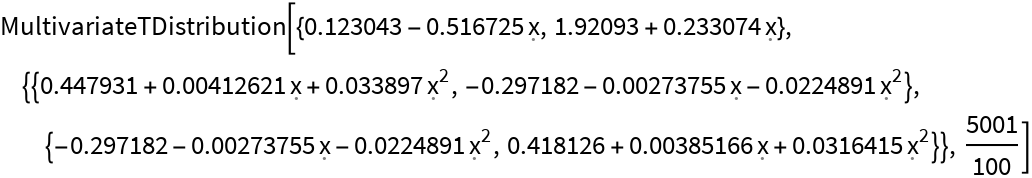Visualize the joint distribution as an animation:

 In:=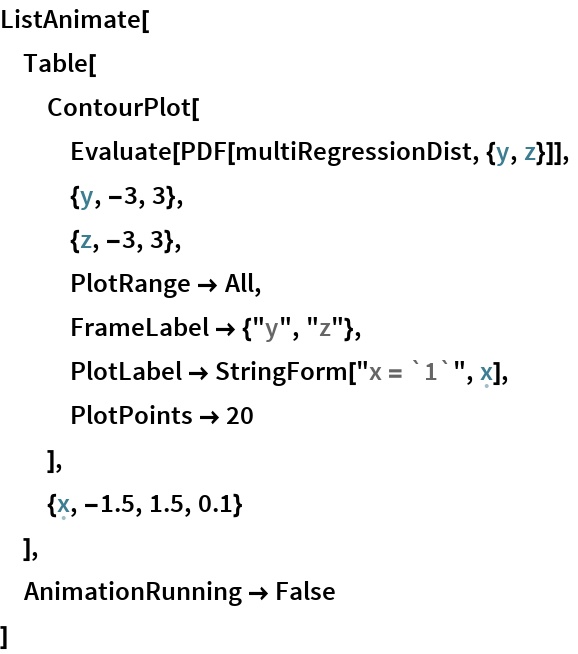Out=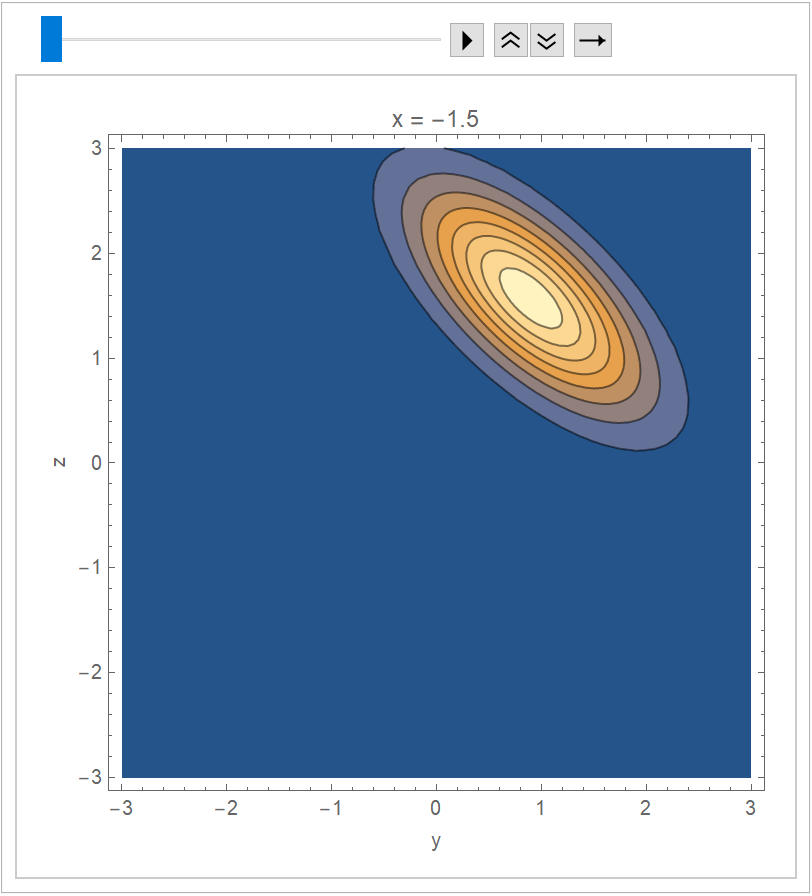### Options

#### IncludeConstantBasis

Just like LinearModelFit, BayesianLinearRegression constructs a model with bias term by default:

 In:=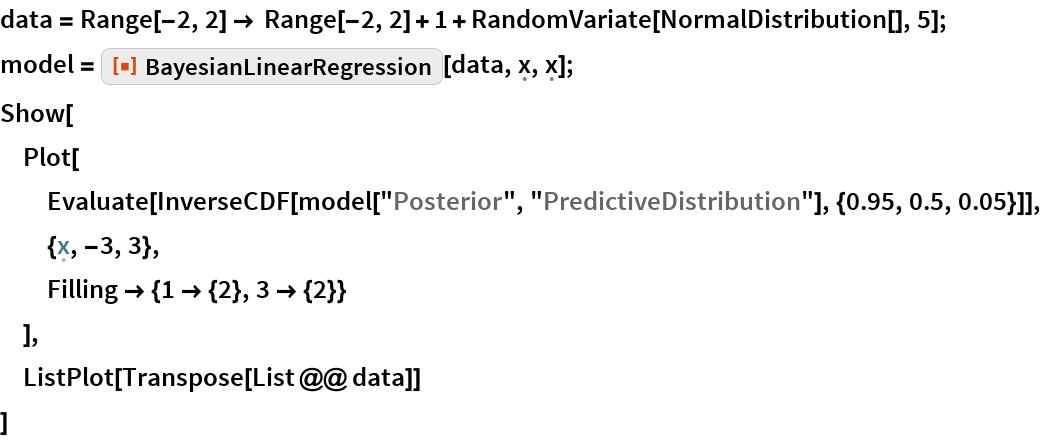Out=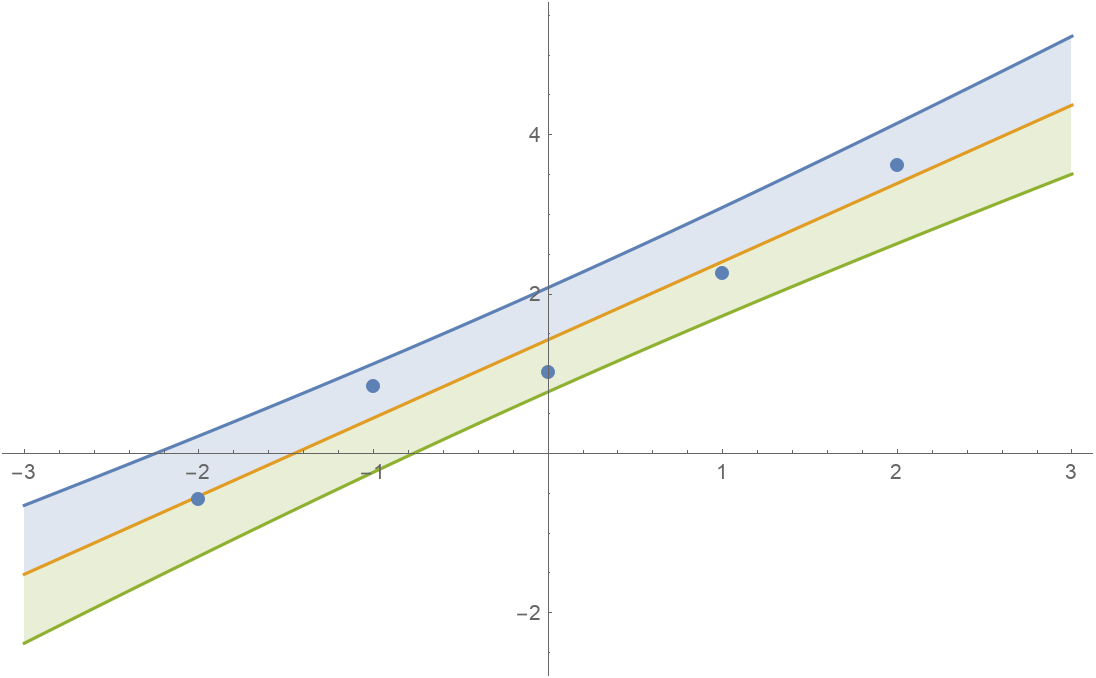Force the intercept to be zero:

 In:=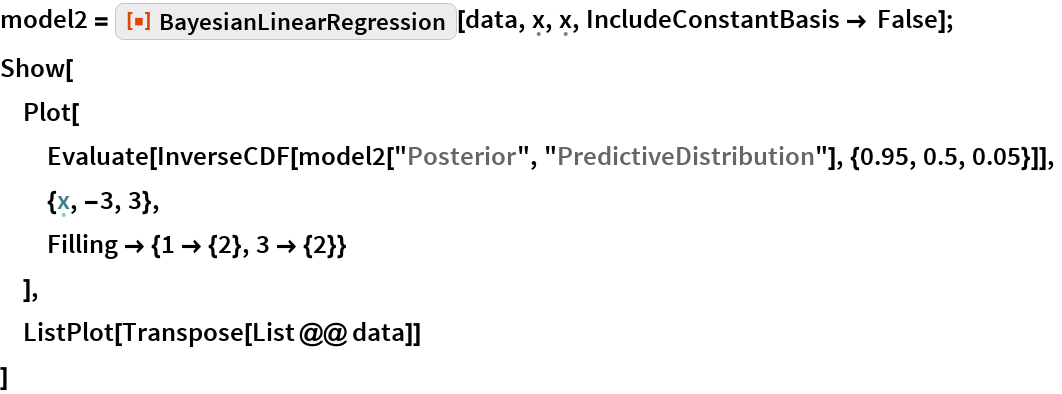Out=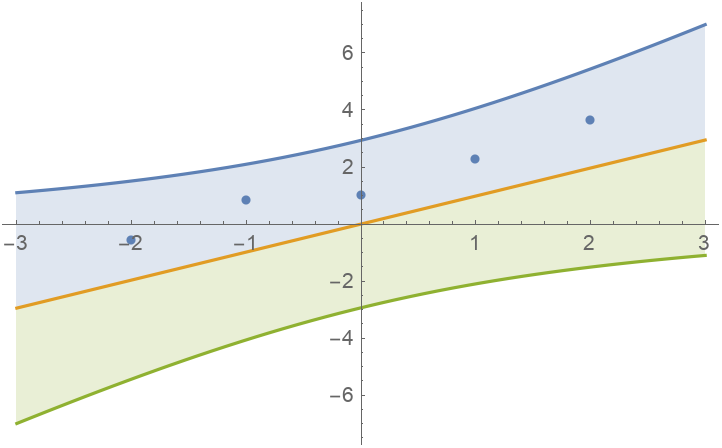#### “PriorParameters”

The prior can be specified in the same format as the parameter outputs of the Bayesian linear regression. This can be used to update a model with new observations. To illustrate this, generate some test data and divide the dataset into 2 parts:

 In:=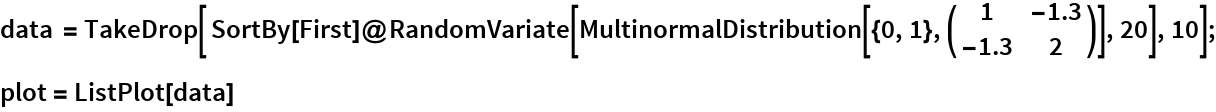Out=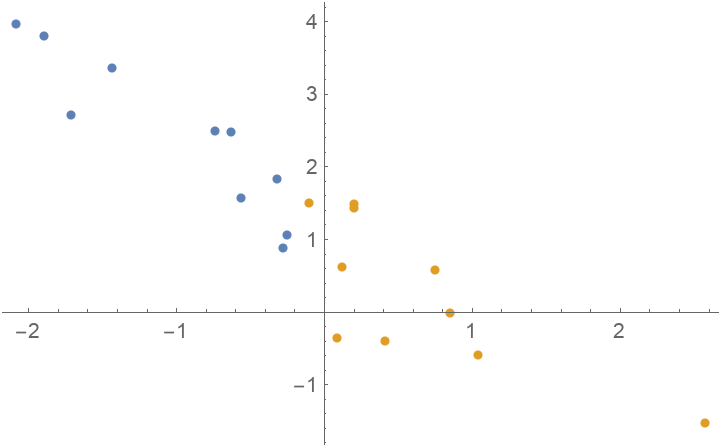Fit the first half of the data:

 In:=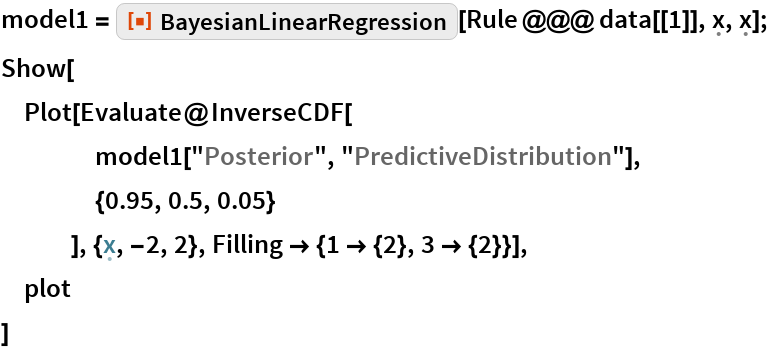Out=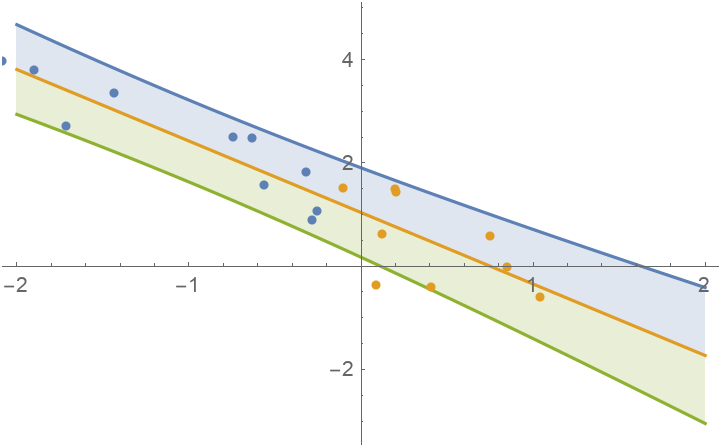Use the posterior parameters of model1 as the prior to update the model with the second half of the dataset:

 In:=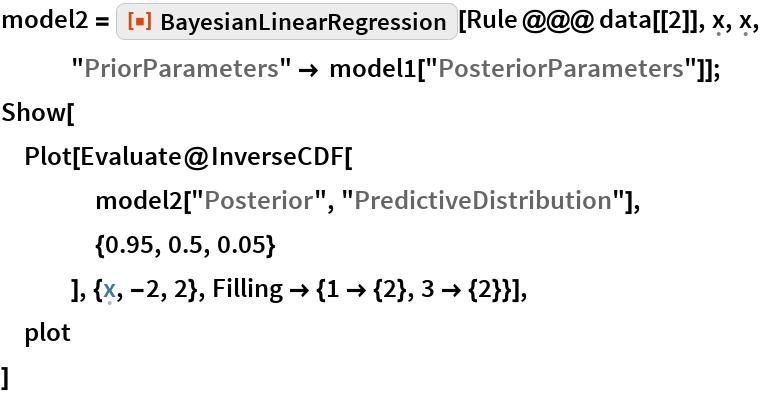Out=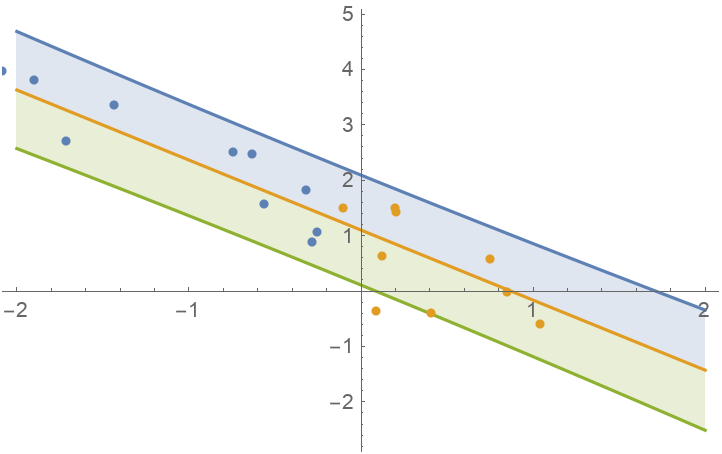The result is exactly the same as when you would fit all of the data in a single step:

 In:=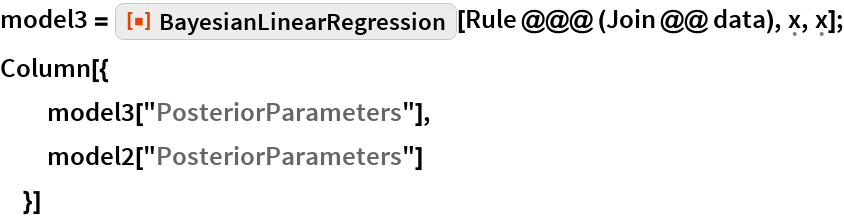Out=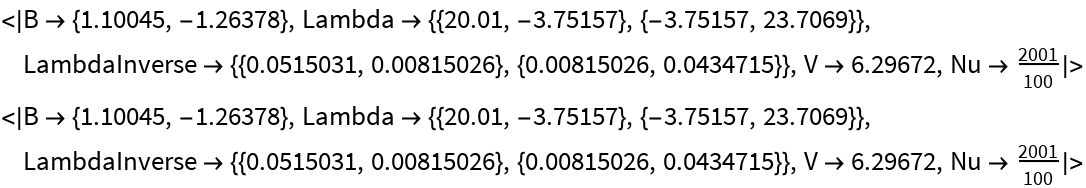#### “IncludeFunctions”

When "IncludeFunctions"True, the returned association contains the key "Functions". This key holds the functions that can be used to generate distributions from arbitrary values of hyperparameters:

 In:=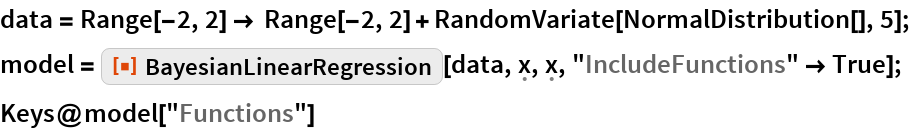Out=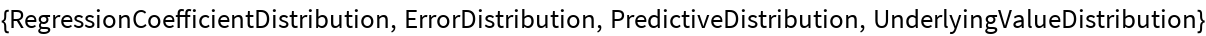The posterior distribution is obtained from the predictive distribution function:

 In:=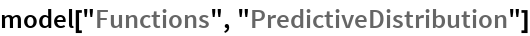Out=Apply it to the posterior parameters:

 In:=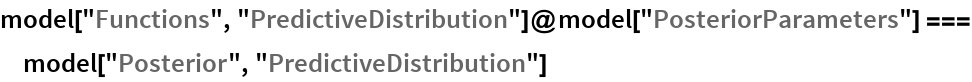Out=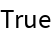### Applications

Here is a dataset where it is unclear if the fit should be first or second order:

 In:=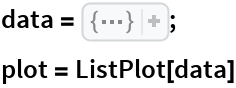Out=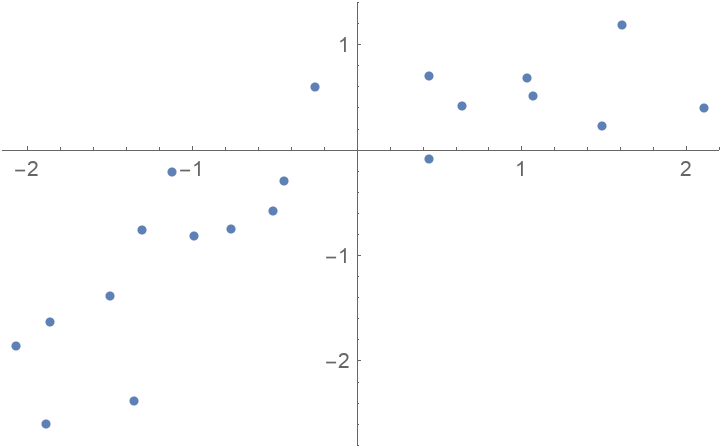Fit the data with polynomials up to fourth degree, rank the log-evidences and inspect them:

 In:=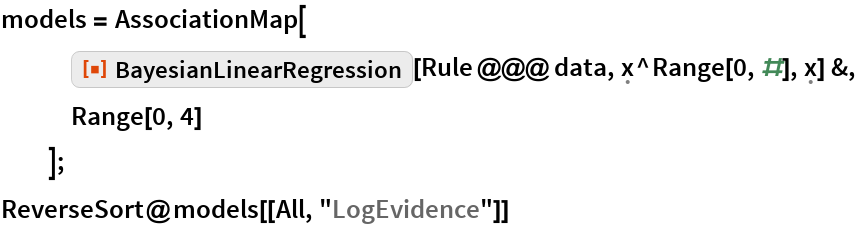Out=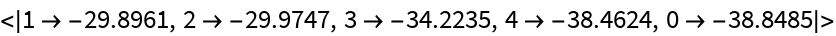Calculate the weights for each fit:

 In:=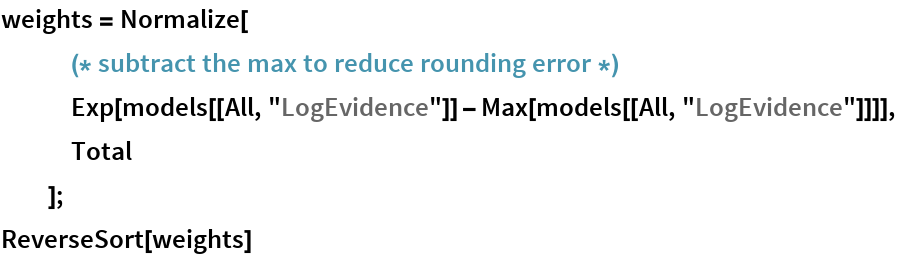Out=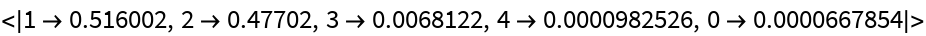Because the weights for the first and second order fits are so close, the correct Bayesian treatment is to include both in the final regression. Define a mixture of posterior predictive distributions:

 In:=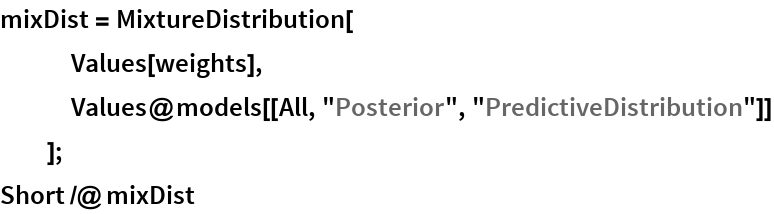Out=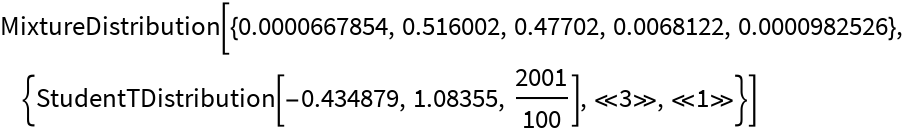Compare the mixture fit to the individual polynomial regressions:

 In:=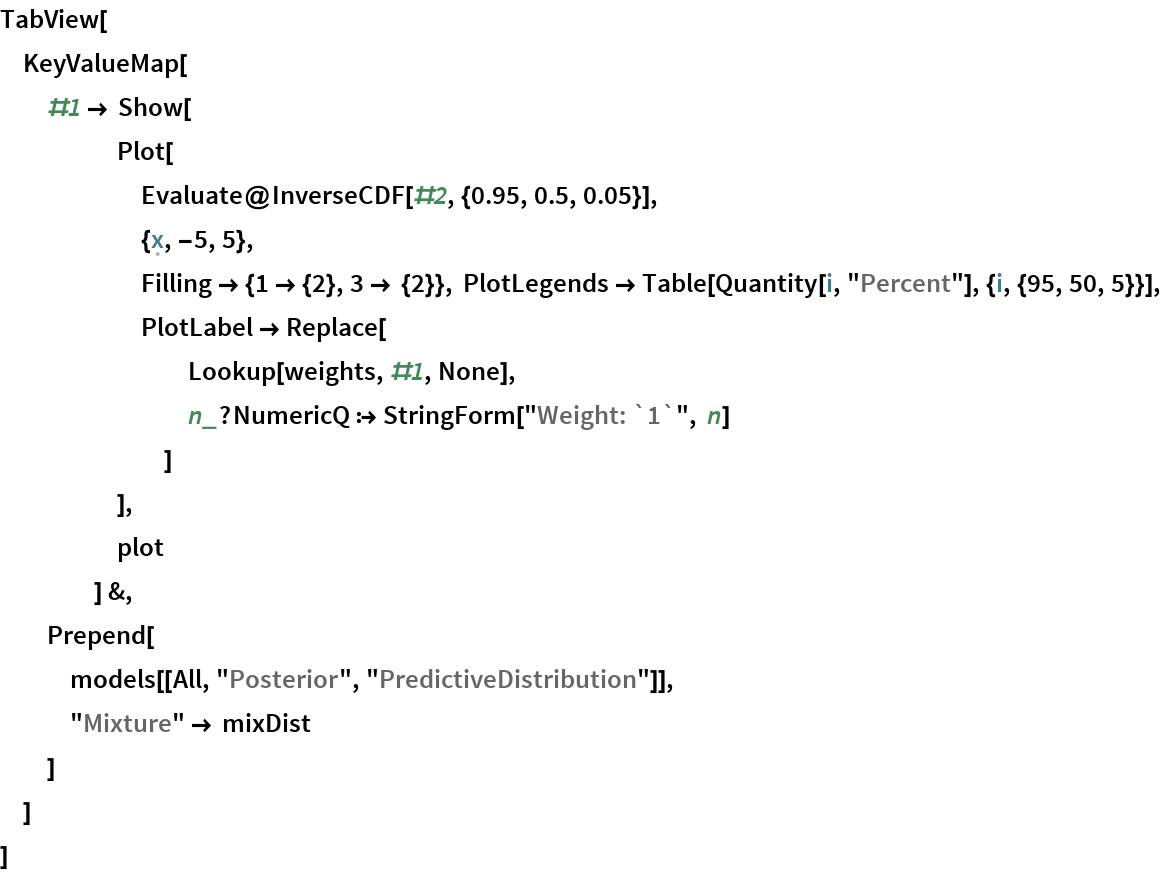Out=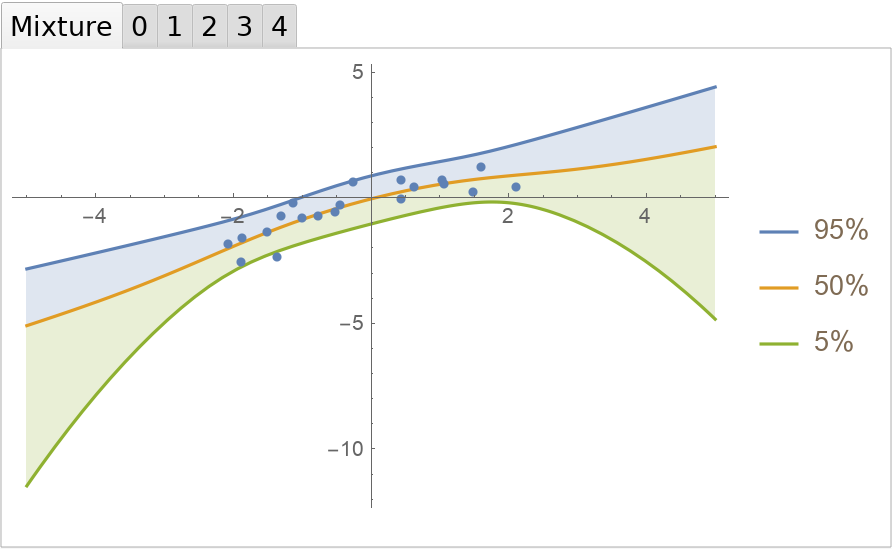### Neat Examples

Symbolically calculate the predictive posterior after a single observation for any prior:

 In:=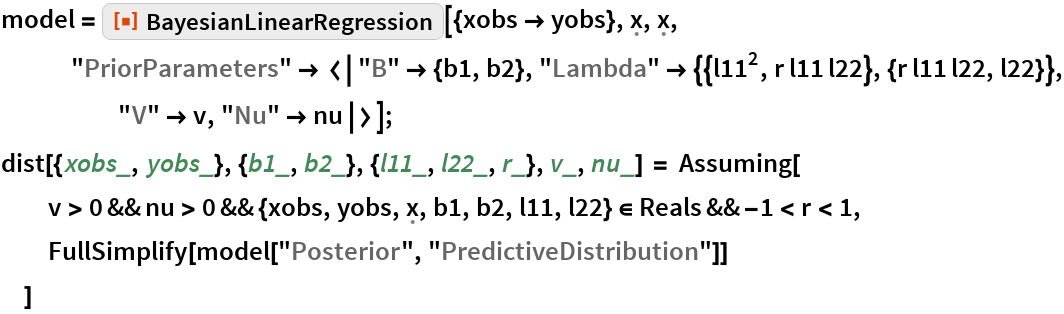Out=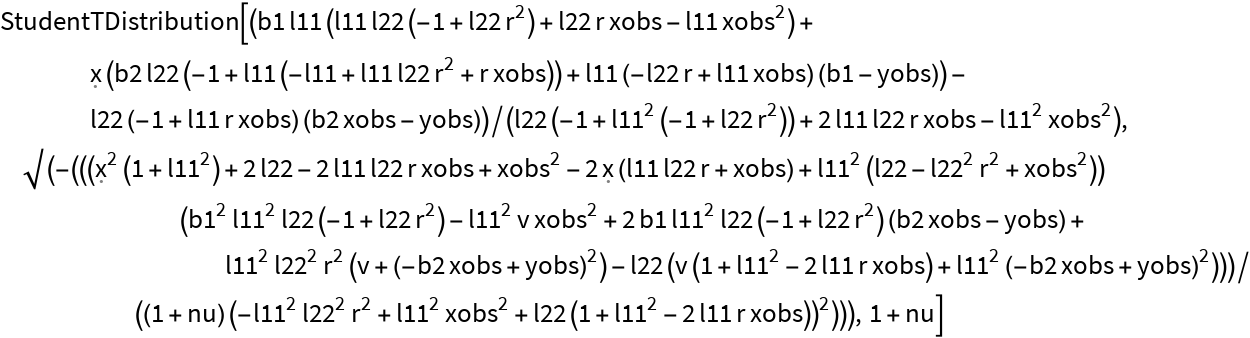Visualize the prediction bands:

 In:=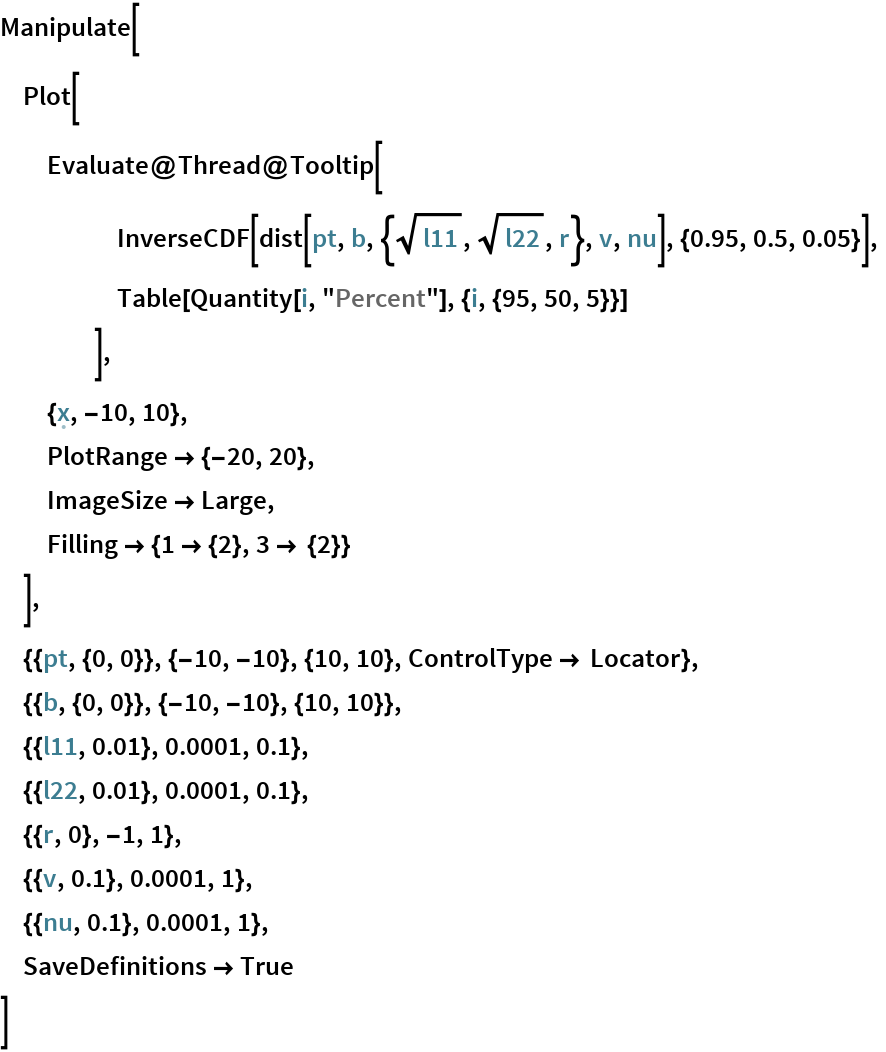Out=